Courses

# RD Sharma Solutions - Chapter 1 - Rational Numbers (Ex -1. 7), Class 8, Maths Class 8 Notes | EduRev

## RD Sharma Solutions for Class 8 Mathematics

Created by: Abhishek Kapoor

## Class 8 : RD Sharma Solutions - Chapter 1 - Rational Numbers (Ex -1. 7), Class 8, Maths Class 8 Notes | EduRev

The document RD Sharma Solutions - Chapter 1 - Rational Numbers (Ex -1. 7), Class 8, Maths Class 8 Notes | EduRev is a part of the Class 8 Course RD Sharma Solutions for Class 8 Mathematics.
All you need of Class 8 at this link: Class 8

Question 1: Divide:

(i) 1 by 1/2
(ii) 5 by −5/7(iii) −3/4 by 9/−16
(iv) −7/8 by −21/16
(v) 7/−4 by 63/64
(vi) 0 by −7/5
(vii) −3/4 by −6
(viii) 2/3 by −7/12
(ix) −4 by −3/5
(x) −3/13 by −4/65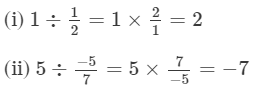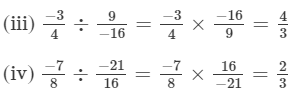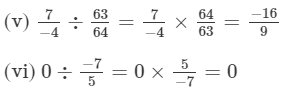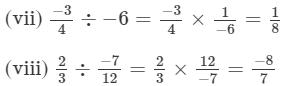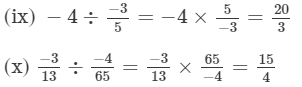Question 2: Find the value and express as a rational number in standard form: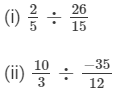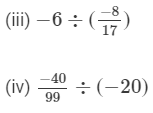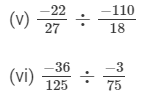####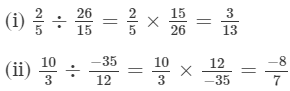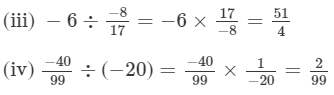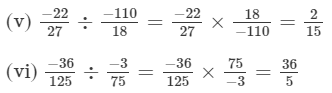Question 3: The product of two rational numbers is 15. If one of the numbers is −10, find the other.

Let the other number be x.

∴ x×(−10)=15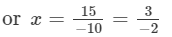So, the other number is -3/2.
Question 4: The product of two rational numbers is -8/9. If one of the numbers is -4/15, find the other.

Answer 4: Let the other number be x.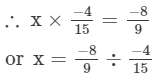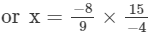or x= 10/3

Thus, the other number is 10/3.
Question 5: By what number should we multiply -1/6-16 so that the product may be -23/9?
Answer 5: Let the number be x.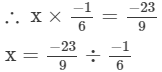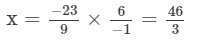Therefore, the other number is 46/3.

Question 6: By what number should we multiply -15/28-1528 so that the product may be -5/7?-57?

Answer 6: Let the other number be x.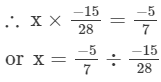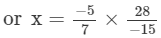or x= 4/3

Thus, the other number is 4/3.
Question 7: By what number should we multiply -8/13 so that the product may be 24?

Answer 7: Let the number be x.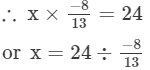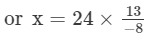or x=39

Thus, the number is −39.
Question 8: By what number should -3/434 be multiplied in order to produce 2/3?23?
Let the other number that should be multiplied with -3/4 to produce2/3 be x.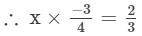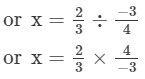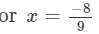Thus, the number is -8/9.

Question 9: Find (x + y) ÷ (x − y), if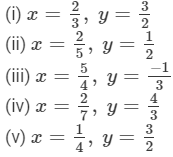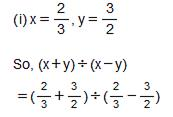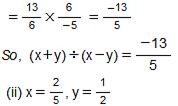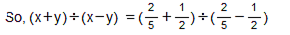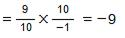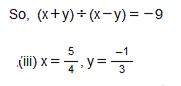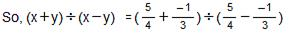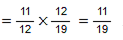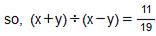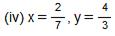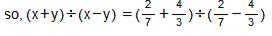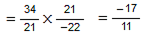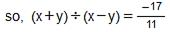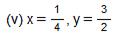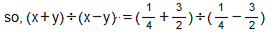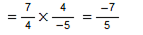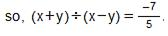Question 10: The cost of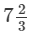metres of rope is Rs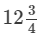. Find its cost per metre.

Answer 10:  The cost ofmetres of rope is Rs.
Cost per metre =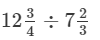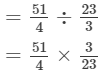= 153/92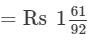Question 11: The cost of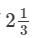metres of cloth is Rs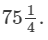Find the cost of cloth per metre.
Answer 11:  The cost ofmetres of cloth is Rs.∴ Cost per metre
=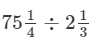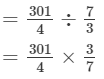= 129/4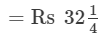Thus,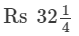or Rs 32.25 is the cost of cloth per metre.
Question 12: By what number should -33/16 be divided to get -11/4?
Answer 12: Let the number be x.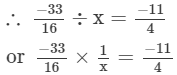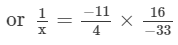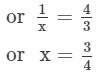Thus, the number is 3/4.

Question 13: Divide the sum of -13/5-135 and 12/7127 by the product of -31/7 and -1/2.-317 and -12.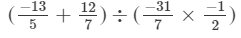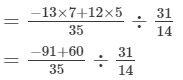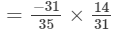= -2/5

Question 14: Divide the sum of 65/12 and 12/7 by their difference.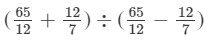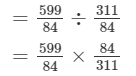= 599/311
Question 15: If 24 trousers of equal size can be prepared in 54 metres of cloth, what length of cloth is required for each trouser?

Answer 15: Cloth needed to prepare 24 trousers=54 m
∴ Length of the cloth required for each trousers= 54÷24= 54/24 = 9/4 m =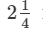metres

91 docs

,

,

,

,

,

,

,

,

,

,

,

,

,

,

,

,

,

,

,

,

,

,

,

,

,

,

,

;